Case Based Questions Test: Arithmetic Progressions

# Case Based Questions Test: Arithmetic Progressions

Test Description

## 15 Questions MCQ Test Mathematics (Maths) Class 10 | Case Based Questions Test: Arithmetic Progressions

Case Based Questions Test: Arithmetic Progressions for Class 10 2022 is part of Mathematics (Maths) Class 10 preparation. The Case Based Questions Test: Arithmetic Progressions questions and answers have been prepared according to the Class 10 exam syllabus.The Case Based Questions Test: Arithmetic Progressions MCQs are made for Class 10 2022 Exam. Find important definitions, questions, notes, meanings, examples, exercises, MCQs and online tests for Case Based Questions Test: Arithmetic Progressions below.
Solutions of Case Based Questions Test: Arithmetic Progressions questions in English are available as part of our Mathematics (Maths) Class 10 for Class 10 & Case Based Questions Test: Arithmetic Progressions solutions in Hindi for Mathematics (Maths) Class 10 course. Download more important topics, notes, lectures and mock test series for Class 10 Exam by signing up for free. Attempt Case Based Questions Test: Arithmetic Progressions | 15 questions in 30 minutes | Mock test for Class 10 preparation | Free important questions MCQ to study Mathematics (Maths) Class 10 for Class 10 Exam | Download free PDF with solutions
 1 Crore+ students have signed up on EduRev. Have you?
Case Based Questions Test: Arithmetic Progressions - Question 1

### Read the following text and answer the following questions on the basis of the same: Amit has a packet of Candies. It consists of 288 candies. He arranges the candies in a way that first row contains 3 candies, second row has 5 and third row has 7 and so on.Q. How many candies are there in the last row?

Detailed Solution for Case Based Questions Test: Arithmetic Progressions - Question 1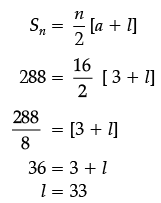Case Based Questions Test: Arithmetic Progressions - Question 2

### Read the following text and answer the following questions on the basis of the same: Amit has a packet of Candies. It consists of 288 candies. He arranges the candies in a way that first row contains 3 candies, second row has 5 and third row has 7 and so on.Q. If Amit want to make 12 rows, then how many total candies will be placed by him with same arrangement.

Detailed Solution for Case Based Questions Test: Arithmetic Progressions - Question 2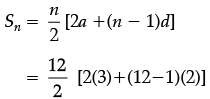= 6 [6 + 22]

= 148

Case Based Questions Test: Arithmetic Progressions - Question 3

### Read the following text and answer the following questions on the basis of the same: Amit has a packet of Candies. It consists of 288 candies. He arranges the candies in a way that first row contains 3 candies, second row has 5 and third row has 7 and so on.Q. How many rows are there?

Detailed Solution for Case Based Questions Test: Arithmetic Progressions - Question 3 The A.P. is 3, 5, 7, ................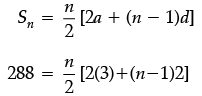⇒ 288 = n [3+(n−1)]

⇒ 288 = n2 + 2n

⇒ n2 + 2n − 288 = 0

⇒ n2 + 18n − 16n − 288 = 0

⇒ n(n + 18) − 16(n + 18) = 0

⇒ (n + 18)(n − 16) = 0

⇒ n = 16

Case Based Questions Test: Arithmetic Progressions - Question 4

Read the following text and answer the following questions on the basis of the same: Amit has a packet of Candies. It consists of 288 candies. He arranges the candies in a way that first row contains 3 candies, second row has 5 and third row has 7 and so on.

Q. Find the difference in the candies placed in the 10th and 15th row.

Detailed Solution for Case Based Questions Test: Arithmetic Progressions - Question 4 a15 − a10 = a + 14d − a − 9d = 5d = 5(2) = 10
Case Based Questions Test: Arithmetic Progressions - Question 5

Read the following text and answer the following questions on the basis of the same: Amit has a packet of Candies. It consists of 288 candies. He arranges the candies in a way that first row contains 3 candies, second row has 5 and third row has 7 and so on.

Q. Is there any row which contains 28 candies?

Detailed Solution for Case Based Questions Test: Arithmetic Progressions - Question 5 No, this is not possible if we see rows are having only odd number of candies.
Case Based Questions Test: Arithmetic Progressions - Question 6

Read the following text and answer the following questions on the basis of the same: In a potato race, a bucket is placed at the starting point, which is 5 m from the first potato and the other potatoes are placed 3 m apart in a straight line. There are ten potatoes in the line (see figures).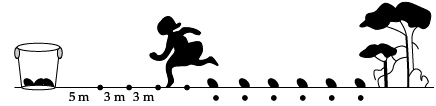A competitor starts from the bucket, picks up the nearest potato, runs back with it, drops it in the bucket, runs back to pick up the next potato, runs to the bucket to drop it in, and she continues in the same way until all the potatoes are dropped in the bucket.

Q. What is the distance run to pick up the 2nd potato?
Detailed Solution for Case Based Questions Test: Arithmetic Progressions - Question 6 Distance run to pick up the 2nd potato = 2 × (5 + 3) = 16 m
Case Based Questions Test: Arithmetic Progressions - Question 7

Read the following text and answer the following questions on the basis of the same: In a potato race, a bucket is placed at the starting point, which is 5 m from the first potato and the other potatoes are placed 3 m apart in a straight line. There are ten potatoes in the line (see figures).A competitor starts from the bucket, picks up the nearest potato, runs back with it, drops it in the bucket, runs back to pick up the next potato, runs to the bucket to drop it in, and she continues in the same way until all the potatoes are dropped in the bucket.

Q. What is the distance run to pick up the 4th potato?

Detailed Solution for Case Based Questions Test: Arithmetic Progressions - Question 7 Distance run to pick up the

4th potato = 2 × (5 + 3 + 3 + 3)

= 28 m.

Case Based Questions Test: Arithmetic Progressions - Question 8

Read the following text and answer the following questions on the basis of the same: In a potato race, a bucket is placed at the starting point, which is 5 m from the first potato and the other potatoes are placed 3 m apart in a straight line. There are ten potatoes in the line (see figures).A competitor starts from the bucket, picks up the nearest potato, runs back with it, drops it in the bucket, runs back to pick up the next potato, runs to the bucket to drop it in, and she continues in the same way until all the potatoes are dropped in the bucket.

Q. What is distance run to pick up the 1st potato?

Detailed Solution for Case Based Questions Test: Arithmetic Progressions - Question 8 Distance run to pick up the 1st potato = 2 × 5 = 10 m
Case Based Questions Test: Arithmetic Progressions - Question 9

Read the following text and answer the following questions on the basis of the same: In a potato race, a bucket is placed at the starting point, which is 5 m from the first potato and the other potatoes are placed 3 m apart in a straight line. There are ten potatoes in the line (see figures).A competitor starts from the bucket, picks up the nearest potato, runs back with it, drops it in the bucket, runs back to pick up the next potato, runs to the bucket to drop it in, and she continues in the same way until all the potatoes are dropped in the bucket.

Q. What is the distance run to pick up the 3rd potato?
Detailed Solution for Case Based Questions Test: Arithmetic Progressions - Question 9 Distance run to pick up the 3rd potato = 2 × (5 + 3 + 3) = 22 m.
Case Based Questions Test: Arithmetic Progressions - Question 10

Read the following text and answer the following questions on the basis of the same: In a potato race, a bucket is placed at the starting point, which is 5 m from the first potato and the other potatoes are placed 3 m apart in a straight line. There are ten potatoes in the line (see figures).A competitor starts from the bucket, picks up the nearest potato, runs back with it, drops it in the bucket, runs back to pick up the next potato, runs to the bucket to drop it in, and she continues in the same way until all the potatoes are dropped in the bucket.

Q. What is the total distance run by the competitor?
Detailed Solution for Case Based Questions Test: Arithmetic Progressions - Question 10 The sequence of distances is 10, 16, 22, 28.

a2 – a1 = 16 – 10 = 6

a3 – a2 = 22 – 16 = 6

a4 – a3 = 28 – 22 = 6

a2 – a1 = a3 – a2 = a2 – a1

∴ The sequence forms an A.P.

Here a = 10, d = a2 – a1= 16 – 10 = 6, n = 10

Now, total distance run by competitor = S10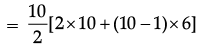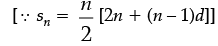= 5[20 + 9 × 6]

= 5[20 + 54]

= 5 × 74

= 370 m

Case Based Questions Test: Arithmetic Progressions - Question 11

Read the following text and answer the following questions.

A manufacturer of TV sets produced 600 sets in the third year and 700 sets in the seventh year, assuming that the production increases uniformly by a fixed number every year.

Q. Find the production in the 10th year.

Detailed Solution for Case Based Questions Test: Arithmetic Progressions - Question 11 a10 = a + 9d = 550 + 9(25) = 550 + 225 = 775
Case Based Questions Test: Arithmetic Progressions - Question 12

Read the following text and answer the following questions.

A manufacturer of TV sets produced 600 sets in the third year and 700 sets in the seventh year, assuming that the production increases uniformly by a fixed number every year.

Q. What is the difference between the productions of two consecutive years?

Detailed Solution for Case Based Questions Test: Arithmetic Progressions - Question 12 The difference between the productions of two consecutive years is 25.
Case Based Questions Test: Arithmetic Progressions - Question 13

Read the following text and answer the following questions.

A manufacturer of TV sets produced 600 sets in the third year and 700 sets in the seventh year, assuming that the production increases uniformly by a fixed number every year.

Q. Find the production in the first year?

Detailed Solution for Case Based Questions Test: Arithmetic Progressions - Question 13 Let a be the production in first year and d be the difference between the productions of two consecutive years.

a3 = a + 2d = 600 …(i)

a7 = a + 6d = 700 …(ii)

Subtracting (i) from (ii),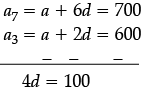d = 25

Put value of d in (i), we get

a = 550

Case Based Questions Test: Arithmetic Progressions - Question 14

Read the following text and answer the following questions.

A manufacturer of TV sets produced 600 sets in the third year and 700 sets in the seventh year, assuming that the production increases uniformly by a fixed number every year.

Q. Find the total production in the first 7 years.

Detailed Solution for Case Based Questions Test: Arithmetic Progressions - Question 14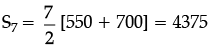Case Based Questions Test: Arithmetic Progressions - Question 15

Read the following text and answer the following questions.

A manufacturer of TV sets produced 600 sets in the third year and 700 sets in the seventh year, assuming that the production increases uniformly by a fixed number every year.

Q. If they produced 600 sets in the year 2010. Then in what years their production will be 1000?

Detailed Solution for Case Based Questions Test: Arithmetic Progressions - Question 15 an = a + (n – 1)d

1000 = 600 +(n – 1) 25

400 = (n – 1) 25

16 = (n – 1)

17 = n

Therefore, in the year 2027.

## Mathematics (Maths) Class 10

53 videos|369 docs|138 tests
 Use Code STAYHOME200 and get INR 200 additional OFF Use Coupon Code
Information about Case Based Questions Test: Arithmetic Progressions Page
In this test you can find the Exam questions for Case Based Questions Test: Arithmetic Progressions solved & explained in the simplest way possible. Besides giving Questions and answers for Case Based Questions Test: Arithmetic Progressions, EduRev gives you an ample number of Online tests for practice

## Mathematics (Maths) Class 10

53 videos|369 docs|138 tests